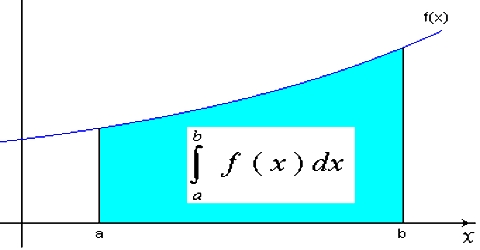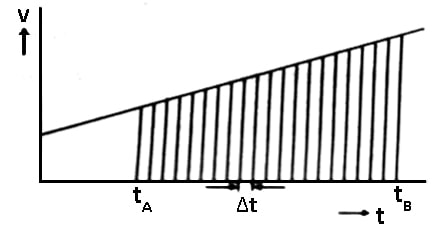Physics

# ﻿Integration in VectorIntegration

Suppose, a body is moving in a particular direction, i.e., it is moving along a straight line. Although the direction of velocity is fixed, its magnitude is varying. Let the magnitude of velocity v is dependent on time t. That means, v is the function of t. is indicated by vt.Let initial time be tA and final time be tB

We will find the distance traveled by the body during this time interval. Let us divide the time interval tB – tA into exceedingly small N number of equal section ∆t. Suppose the time interval from the initial time tA to first small section tA + ∆t is ∆t. This time interval is so small that we can consider that the value of vt is almost constant. Let the constant value of vt be v. If the distance traveled in time ∆t is ∆S1 then we get,

∆S1 = v1∆t

Similarly, for the second, third sections time interval is ∆t and the distance traveled will be respectively ∆S2 = v2∆t and ∆S3 = v3∆t. For the Nth section, distance traveled is ∆SN = vN∆t. Now, distance traveled between the time intervals tA and tB will be the summation ∆S1, ∆S2, ∆S3……., ∆SN.

So, S = ∆S1 + ∆S2 + ∆S3…….+ ∆SN.

or, S = v1∆t + v2∆t + v3∆t + ……… + vN∆t.

or, S = Nn = 1 = Vn ∆t. … … … (1)

It is to be noted that here we have assumed that the value of velocity is constant for small-time Interval ∆t. if the value of v would have been exactly constant, then we could get the more accurate value of the distance from the equation (1). Now as the time interval becomes more and smaller, the value of v will be more and closer to the constant value. We can get the accurate value of distance if ∆t tends to zero and the number of sections N becomes infinity. Then we can write;

S = lim∆t→0 Nn = 1 = Vn ∆t

In calculus, the quantity lim∆t→0 Nn = 1; is written as, tBtA v(t)dt

The quantity tBtA v(t)dt indicates the integration of v(t) from tA to tB with respect to t.

Integration is a type of summation, and the symbol ∑ or ∫ indicates that summation or integration.

So, s = tBtA v(t)dt  … … … (2)

v(t) is called integrand. dt after v(t) indicates that integration is to be done with respect to t. Differentiation and integration are opposite to each other. For example,

d/dt (sin x) = cos x

again, ∫cos x dx = sin x.

That means, if sin x as differentiated we get cos x, on the other hand, if cos x is integrated we get sin x.